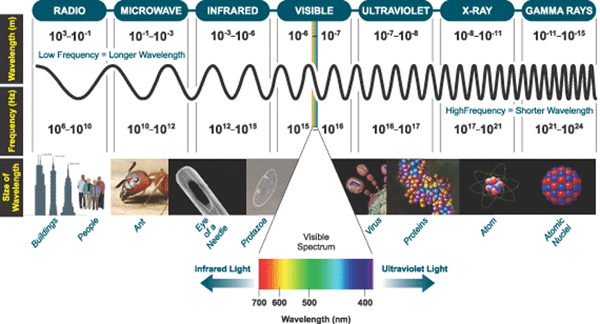Electromagnetic Waves

Electromagnetic waves are a form of energy that consist of vibrating electric and magnetic fields. Electric fields are produced by forces of electric charges, and magnetic fields are produced when electric charges are in motion. When an appliance is plugged in, an electric field is produced around the appliance; when the appliance is turned on and the electrical current is flowing, a magnetic field is produced.

The main natural source of electromagnetic radiation is the sun. Natural electromagnetic energy (i.e., sunlight) is necessary for photosynthesis in plants. Man-made sources, however, account for most of the electromagnetic radiation in our environment. With the proliferation of new technological devices in our home and workplace we are all exposed to electromagnetic radiation daily. Everyday household electrical devices such as hairdryers, electrical ovens, fluorescent lights, microwave ovens, stereos, mobile phones, and computers and the transmitters that support these items emit electrical and magnetic fields of varying intensities.

Measurement of electromagnetic fields

Electric and magnetic fields are characterized by wavelength and by frequency. Wavelength is the distance that a wave travels in one cycle of oscillation and is measured in metres. The frequency is measured by the number of cycles per second, and the unit of measurement is the Hertz (Hz). One cycle per second equals one Hertz. One kilohertz (kHz) is 1,000 Hz; one megahertz (MHz) is 1 million Hz; one gigahertz (GHz) is 1 billion Hz. The frequency of a wave is inversely related to its length by a simple mathematical formula: frequency times wavelength = the speed of light. Hence, the higher the frequency, the shorter is the wavelength. At 50 Hz the wavelengths are 6,000 Km, and at 100 MHz they are 3 meters. The electromagnetic spectrum is divided into ionizing and non-ionizing bands based on how the wave interacts with biological tissue. Ionization involves removal of electrons from their normal positions in atoms and molecules, and can damage tissues, including genetic material. The ionizing portion of the EM spectrum is made up of ultraviolet light, gamma rays and X-rays. These have very short wavelengths, very high frequencies, and very high intensities. The non-ionizing portion includes the extremely low frequency (ELF) band, radio waves and microwaves in the radio frequency (RF) communications band, and infrared and visible light. Radio waves are usually defined as those in the range 30 kHz to 300 GHz. Microwaves are a subset of radio waves.

Electromagnetic SpectrumOther terms used in measurement of EMF are shown in the table below.

 Term Unit Comment Frequency Hertz (Hz) Number of times per second a wave goes through its maximum value Electric Field Strength Volts/metre (V/m) Signified by “E” Magnetic Field Strength Amperes/metre (A/m) Signified by “H” Magnetic Flux Density Tesla (T), or Gauss (G), where 10,000 G = 1T Signified by “B”

At low frequencies such as power line frequencies, magnetic flux units are more commonly used; so the magnetic field is expressed usually in mG (milliGauss) or lT (microTesla) with 1 mG = 10 lT. The earth produces a static magnetic field, which ranges from 350 to 700 mG, over the surface of the planet, and which varies slightly according to daily and yearly rhythms. There is also a naturally occurring electrical field associated with varying charge differences between the earth and the atmosphere.

At radio frequencies the measurements used depend on whether the source of energy is remote, or near to the person exposed. Power density is used in “far-field” situations i.e. when a measurement is taken more than several wavelengths distance from a RF source. Far-field sources include TV, radio and cellular phone transmission towers and antennae. Power density is defined as the rate of energy flow through a given surface area. It is measured in watts per square metre (W/m2, or in mW/m² or /cm²). One mW equals 0.001 watt of power and one µW equals 0.000001 watt, so that 1mW/cm² = 1,000 µW/ cm² or 10 W/m².

Near-field exposure, on the other hand, is the exposure in close proximity to an EMF source. Examples of near-field radiation sources, to the user or consumer, include cellular phones, radar guns, and some household appliances. For near-field exposures, the Specific Absorption Rate (SAR) is used. It is defined as the rate of energy absorption per unit mass, and is expressed in units of W/kg. SAR measures how much RF energy the body absorbs. The measurement is difficult, and is usually derived from measures of power density. SAR is discussed in a section under “wireless phones” of the web site.